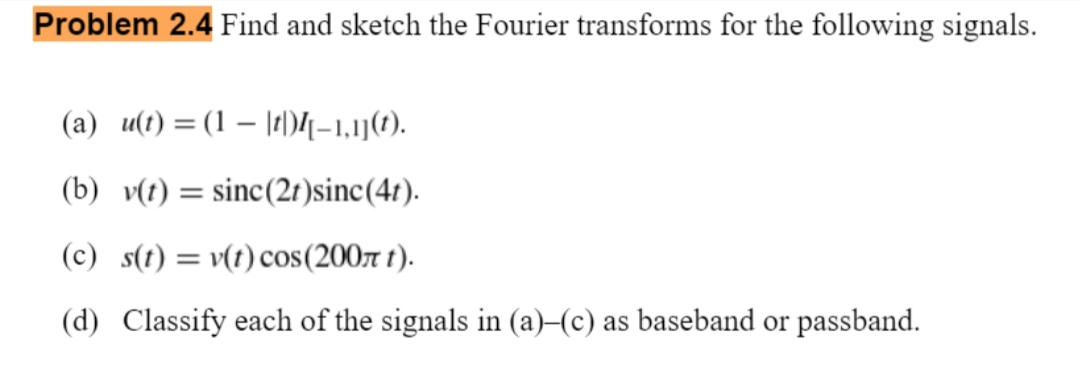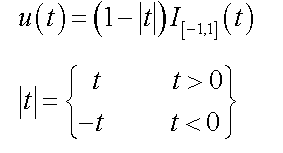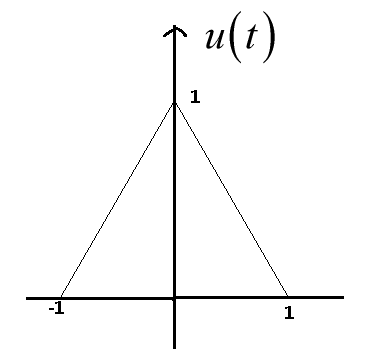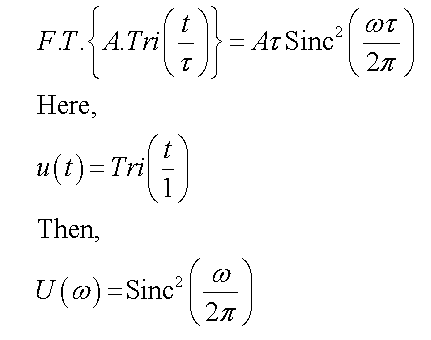# Problem 2.4 Find and sketch the Fourier transforms for the following signals.(a) u(t) = (1 – |t)4-1,1j().(b) v(t) = sinc(2t )sinc(4r).(c) s(t) = v(t) cos(200r t).(d) Classify each of the signals in (a)–(c) as baseband or passband.

Question
39 viewshelp_outlineImage TranscriptioncloseProblem 2.4 Find and sketch the Fourier transforms for the following signals. (a) u(t) = (1 – |t)4-1,1j(). (b) v(t) = sinc(2t )sinc(4r). (c) s(t) = v(t) cos(200r t). (d) Classify each of the signals in (a)–(c) as baseband or passband. fullscreen
check_circle

Step 1

We are authorized to answer three subparts at a time, since you have not mentioned which part you are looking for, so we are answering the first three subparts, please repost your question separately for the remaining subpart.

(a).

The expression for the function is given as:Step 2

Sketching the graph for the above function:Step 3

The above signal is the baseband signal and can also be represented in the form of triangular function.

Now evaluating the Fourier transform using its property:...

### Want to see the full answer?

See Solution

#### Want to see this answer and more?

Solutions are written by subject experts who are available 24/7. Questions are typically answered within 1 hour.*

See Solution
*Response times may vary by subject and question.
Tagged in

### Electrical Engineering